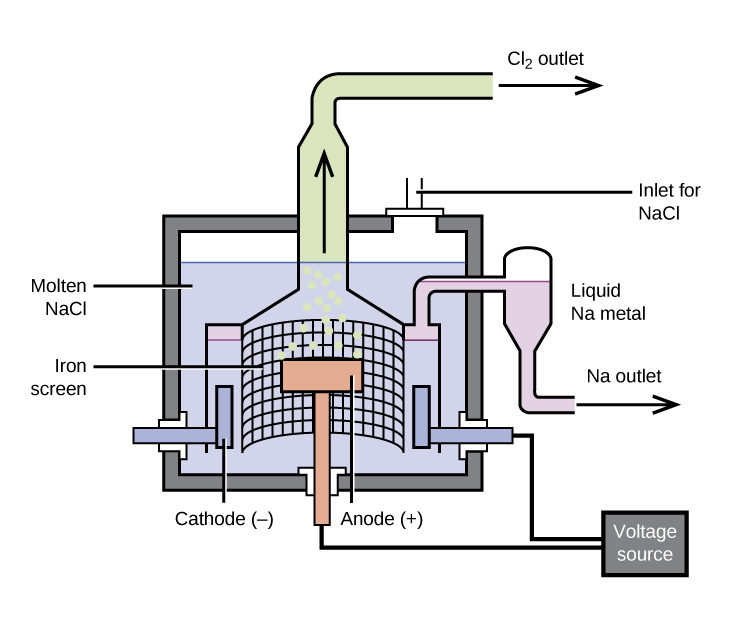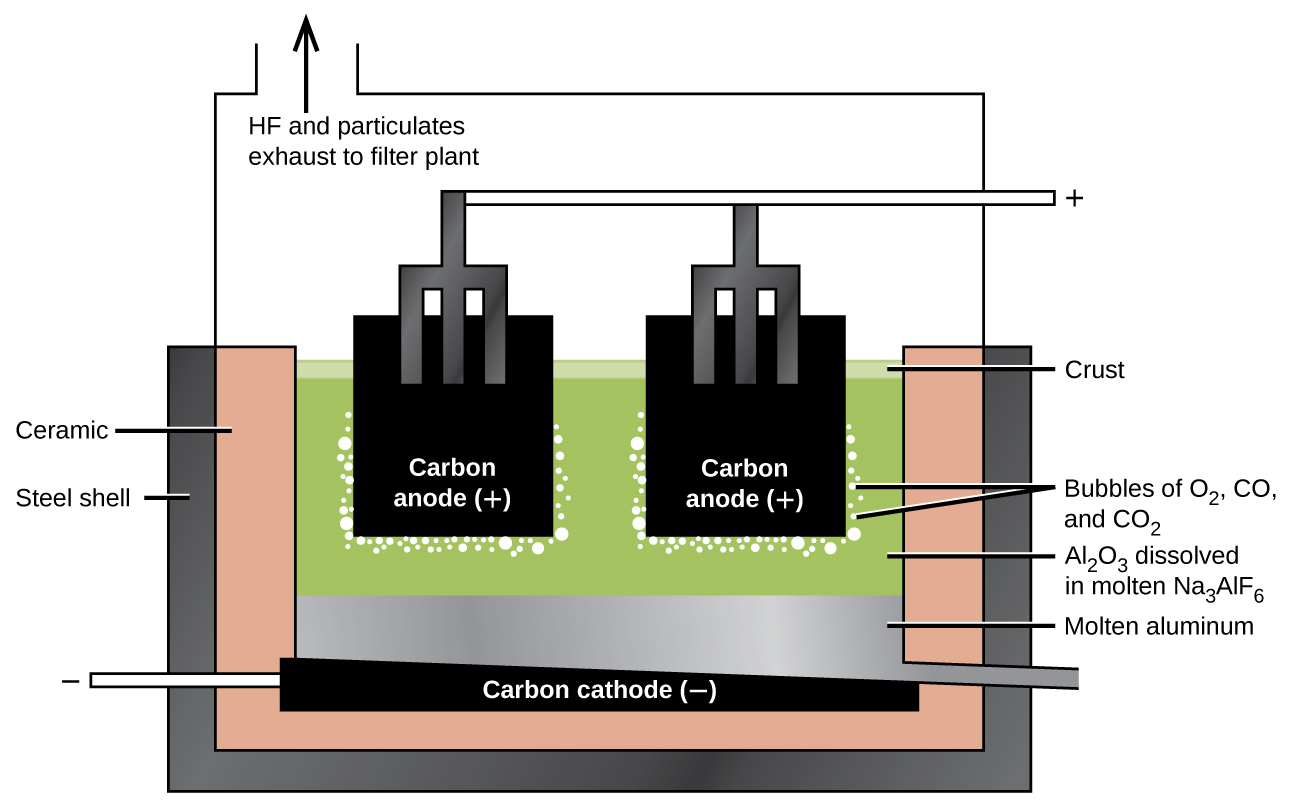# 18.2 Occurrence and preparation of the representative metals  (Page 2/7)

 Page 2 / 7Pure sodium metal is isolated by electrolysis of molten sodium chloride using a Downs cell. It is not possible to isolate sodium by electrolysis of aqueous solutions of sodium salts because hydrogen ions are more easily reduced than are sodium ions; as a result, hydrogen gas forms at the cathode instead of the desired sodium metal. The high temperature required to melt NaCl means that liquid sodium metal forms.

## The preparation of aluminum

The preparation of aluminum utilizes a process invented in 1886 by Charles M. Hall , who began to work on the problem while a student at Oberlin College in Ohio. Paul L. T. Héroult discovered the process independently a month or two later in France. In honor to the two inventors, this electrolysis cell is known as the Hall–Héroult cell    . The Hall–Héroult cell is an electrolysis cell for the production of aluminum. [link] illustrates the Hall–Héroult cell.

The production of aluminum begins with the purification of bauxite, the most common source of aluminum. The reaction of bauxite, AlO(OH), with hot sodium hydroxide forms soluble sodium aluminate, while clay and other impurities remain undissolved:

$\text{AlO}\left(\text{OH}\right)\left(s\right)+\text{NaOH}\left(aq\right)+{\text{H}}_{2}\text{O}\left(l\right)\phantom{\rule{0.2em}{0ex}}⟶\phantom{\rule{0.2em}{0ex}}\text{Na}\left[\text{Al}{\left(\text{OH}\right)}_{4}\right]\left(aq\right)$

After the removal of the impurities by filtration, the addition of acid to the aluminate leads to the reprecipitation of aluminum hydroxide:

$\text{Na}\left[\text{Al}{\left(\text{OH}\right)}_{4}\right]\left(aq\right)+{\text{H}}_{3}{\text{O}}^{\text{+}}\left(aq\right)\phantom{\rule{0.2em}{0ex}}⟶\phantom{\rule{0.2em}{0ex}}\text{Al}{\left(\text{OH}\right)}_{3}\left(s\right)+{\text{Na}}^{\text{+}}\left(aq\right)+{\text{2H}}_{2}\text{O}\left(l\right)$

The next step is to remove the precipitated aluminum hydroxide by filtration. Heating the hydroxide produces aluminum oxide, Al 2 O 3 , which dissolves in a molten mixture of cryolite, Na 3 AlF 6 , and calcium fluoride, CaF 2 . Electrolysis of this solution takes place in a cell like that shown in [link] . Reduction of aluminum ions to the metal occurs at the cathode, while oxygen, carbon monoxide, and carbon dioxide form at the anode.An electrolytic cell is used for the production of aluminum. The electrolysis of a solution of cryolite and calcium fluoride results in aluminum metal at the cathode, and oxygen, carbon monoxide, and carbon dioxide at the anode.

## The preparation of magnesium

Magnesium is the other metal that is isolated in large quantities by electrolysis. Seawater, which contains approximately 0.5% magnesium chloride, serves as the major source of magnesium. Addition of calcium hydroxide to seawater precipitates magnesium hydroxide. The addition of hydrochloric acid to magnesium hydroxide, followed by evaporation of the resultant aqueous solution, leaves pure magnesium chloride. The electrolysis of molten magnesium chloride forms liquid magnesium and chlorine gas:

${\text{MgCl}}_{2}\left(aq\right)+\text{Ca}{\left(\text{OH}\right)}_{2}\left(aq\right)\phantom{\rule{0.2em}{0ex}}⟶\phantom{\rule{0.2em}{0ex}}\text{Mg}{\left(\text{OH}\right)}_{2}\left(s\right)+{\text{CaCl}}_{2}\left(aq\right)$
$\text{Mg}{\left(\text{OH}\right)}_{2}\left(s\right)+\text{2HCl}\left(aq\right)\phantom{\rule{0.2em}{0ex}}⟶\phantom{\rule{0.2em}{0ex}}{\text{MgCl}}_{2}\left(aq\right)+{\text{2H}}_{2}\text{O}\left(l\right)$
${\text{MgCl}}_{2}\left(l\right)\phantom{\rule{0.2em}{0ex}}⟶\phantom{\rule{0.2em}{0ex}}\text{Mg}\left(l\right)+{\text{Cl}}_{2}\left(g\right)$

Some production facilities have moved away from electrolysis completely. In the next section, we will see how the Pidgeon process leads to the chemical reduction of magnesium.

## Chemical reduction

It is possible to isolate many of the representative metals by chemical reduction    using other elements as reducing agents. In general, chemical reduction is much less expensive than electrolysis, and for this reason, chemical reduction is the method of choice for the isolation of these elements. For example, it is possible to produce potassium, rubidium, and cesium by chemical reduction, as it is possible to reduce the molten chlorides of these metals with sodium metal. This may be surprising given that these metals are more reactive than sodium; however, the metals formed are more volatile than sodium and can be distilled for collection. The removal of the metal vapor leads to a shift in the equilibrium to produce more metal (see how reactions can be driven in the discussions of Le Châtelier’s principle in the chapter on fundamental equilibrium concepts).

give two properties of liquid
what is measurement
is the comparison of an unknown quantity with a fixed quantity of the same kind
How does an element differ from a compound? How are they similar?
an element is an indivisible particles that can take part in a reaction and consist of smaller or tiny particles i.e proton, neutrons and electron while a compound is when two or more element chemically combine together. They are similar when they are homogeneous compound. they take the same rxn.
Yusuf
an element is s chemically pure substance containing a particular type of atoms.. A COMPOUND is a substance containing atoms from different elements..
Inemesit
How to get the Lewis formula of SeCl+3
hi,I'm new here can I join the conversation
EZEA
what is the structural formula for starch
Starch is a mixture (of chemicals) of amylose and amylopectin. Both are macromolecules and polymers. You can search on wikipedia.
Abdelkarim
what is the roles of filter bed
Fathmat
what is the roles of Alu m
Fathmat
what is the roles of chlorine
Fathmat
Roles can be classified or correlate it to different areas: For example: Chlorine can be used in reactions (in industry) to manufacture HCl, which then can be used for other things. Or in swimming pools to kill bacteria. Or as a component in compounds with pharmaceutical roles (drugs). For Al:
Abdelkarim
Its dentisty value is suitable to be used in alloys (mixture of metals) in aircraft bodies. Also, Aluminium foils, Tin cans,.. Some of them are also in Al overhead cables in streets and long roads.
Abdelkarim
what is chemistry
Maxamed
what is the meaning of exceedingly
it is an adverb which means extremely
Rohini
what is atomic chemistry?
Lewis structure for no3
Lewis structure for no3
what is weak acid
It is an acid which partially ionises in water.
Abdelkarim
what is incandescence
Clifton
what makes it glow
Clifton
why is it red, irange and yellow in color
Clifton
hello am new here and I want to join you
Aliyu
hello
Clifton
hi
Aliyu
too
Gillian
hello i am new here please i want to join this group
Paul
Hi, I'm also new here
Salaudeen
Hi
Keeya
hello guys !!
Sourav
what is pressure?
The force applied to suction Area of the body
Ahmed
Matter composed of exceedingly small paticle called atom.
Yushao
questions related to metals
occurrence and preparation of the representatives metals
Regina
list the 20, periodic table and their symbols
hydrogen:h helium;he lithium:l beryllium:be Boron:b Carbon;C Nitrogen:n Oxygen:O FLUORINE:f Neon:n Sodium:s Magnesium:mg Aluminum:a Silicon:s Phosphorus:p Sulphur:s Chlorine:c Argon;a Potassium:p Calcium:c
Benita
Hydrogen, helium, lithium, beryllium, boron, carbon, nitrogen, oxygen, fluorine, neon, sodium, magnesium, aluminium, silicon, phosphorus, sulphur, chlorine, argon, potassium, calcium
Cudjoe
what is a solute
Any substance that is disolved in a liqid solvent to create a solution
Fifa
sorry liquid
Fifa
it's a liquid substance
Fathmat
hello group
Ayomide
is the substance that dissolves in the solvent
Amos
so is HCl ionic compound
No, covalent compound ➡️ molecule. As both H and Cl are non-metals and and form covalent bind by sharing valence e-. But can fully ionice in water forming H+ (a proton, a reason for acidity) and Cl- (anion =Chloride) Hydrogen Chloride is a gas at room; Hydrochloric acid = HCl (aq), dissolved in w
Abdelkarim
Form covalenr bond*
Abdelkarim
The question marks are an emoji in the first sentence is an unread emoji. HCl Covalent compund -> molecule
Abdelkarim
Hi.
Queen
Hi
Calvin
Yh
Cudjoe
yes
Amos
what is chemistry
is the study of composition of substances and the way they behave under different conditions
Amos
how do calculate n1 though n6 any help on understanding the concept
Clifton
is the study of properties of matter and it's component
Grace
where can I get the test bank or mcqs ? any idea ?ByByBy Eddie UnverzagtBy Steve GibbsBy John GabrieliBy OpenStaxBy Cath YuBy Edgar DelgadoBy OpenStaxBy OpenStaxBy OpenStaxBy Ann Schlosser